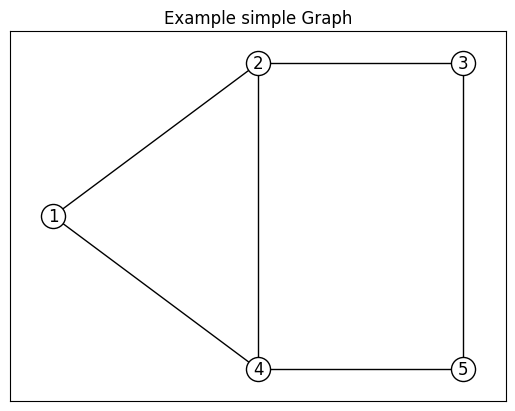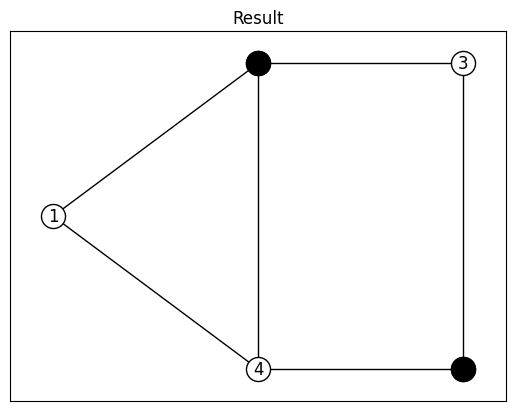# Maximum-Cut¶

Maximum-Cut is the problem of maximizing the weights of edges between different groups when partitioning into two subsets in a given vertex set of graph.

## Simple Example¶

First, let’s we solve the maximum-cut of following simple graph.

data = """5 6
1 2 1
1 4 1
2 3 1
2 4 1
3 5 1
4 5 1
"""

import networkx
import matplotlib.pyplot as plt

# create networkx graph
G = networkx.Graph()
f = iter(data.split("\n"))
N, E = map(int, next(f).split())  # N is the number of vartexes, and E is the number of edges
for e in range(E):
i, j, w = map(int, next(f).split())

# plot the graph
fig, ax = plt.subplots()
pos = {1: (0, 1), 2: (1, 2), 3: (2, 2), 4: (1, 0), 5: (2, 0)}
networkx.draw_networkx(G, pos, with_labels=True, node_color="white", edgecolors="black")
ax.set_title("Example simple Graph")We divide these five vertexes into two groups, $$S$$ and $$T$$. We create and assign a spin variable $$s_i$$ to the vertex $$i$$ of the graph, where spin variable only takes +1 or -1. $$s_i = +1$$ represents vertex $$i$$ is in group $$S$$, and $$s_i = -1$$ represents vertex $$i$$ is in group $$T$$. This gives us that $$(1 - s_i s_j)$$ takes 0 only if vertex $$i$$ and $$j$$ are belong to same group, and takes 1 only if these vertexes are belongs to different groups. Hence, the maximum cut problem is formulated as $$\max \sum_{i < j} w_{i, j} (1 - s_i s_j)$$, where $$w_{i, j}$$ is the weight of edge $$(i, j)$$.

from flopt import *

G = networkx.Graph()
f = iter(data.split("\n"))
N, E = map(int, next(f).split()) # N is the number of vartexes, and E is the number of edges

# create spin variables
s = Variable.array("s", N, cat="Spin", ini_value=1)

# create objective function
obj = 0
for e in range(E):
i, j, w = map(int, next(f).split())
obj += w * (1 - s[i-1] * s[j-1])

# create problem
prob = Problem(sense=Maximize)
prob += obj

# solve
staus, log = prob.solve(solver="Random", timelimit=1)

print("result = ", Value(s))
>>> [-1 1 -1 -1 1]


Plot the result partitioning.

# plot the graph
nodelist = [i+1 for i in range(N) if s[i].value() == 1]
networkx.draw_networkx_nodes(G, pos, nodelist=nodelist, node_color="black", ax=ax)
ax.set_title("Result")## Gset Benchmark¶

Gset is the benchmark the maximize cut problem. We can download the Gset benchmark as follows.

mkdir Gset && cd Gset; for i in {1..81}; do wget http://web.stanford.edu/~yyye/yyye/Gset/G\$1; done

# select problem
file = "./Gset/G11"

from flopt import *

for i in range(n):
yield map(int, next(f).split())

# load problem, and create spin variables and objective function
with open(file, "r") as f:
N, E = map(int, next(f).split())
s = Variable.array("s", N, cat="Spin")
obj = 0.5 * Sum(w * (1 - s[i-1] * s[j-1]) for i, j, w in loader(f, E))

# create Problem
prob = Problem(sense=Maximize)
prob += obj

# select algorithm to search and solve
status, log = prob.solve(solver="Random", timelimit=10, msg=True)


## Convert another formulations¶

We can obtain the data for the another formulation using flopt.convert, for example ising structure.

$$\min - x^T J x - h^T x + C$$

import flopt.convert

ising = flopt.convert.IsingStructure.fromFlopt(prob)
print(ising.J)
print(ising.h)
print(ising.x)


When you have the solution by your algorithm or other applications, you can input the value to the spin variable of flopt.

values = [...]  # solution; list of values
for var, value in zip(ising.x, values):
var.setValue(value)